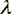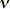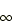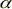Constants Topics: Values Energy Equivalents Searchable Bibliography Background Constants Bibliography Constants, Units & Uncertainty home pageBasis of conversion factors for energy equivalents

Conversion factors for energy equivalents are derived from the following relations:

E = mc2 = hc/= h= kT

1 eV = (e/C) J

1 u = mu = (1/12) m (12C) = 2Rh/(Ar(e)2c)

Eh = 2Rhc =2mec2.